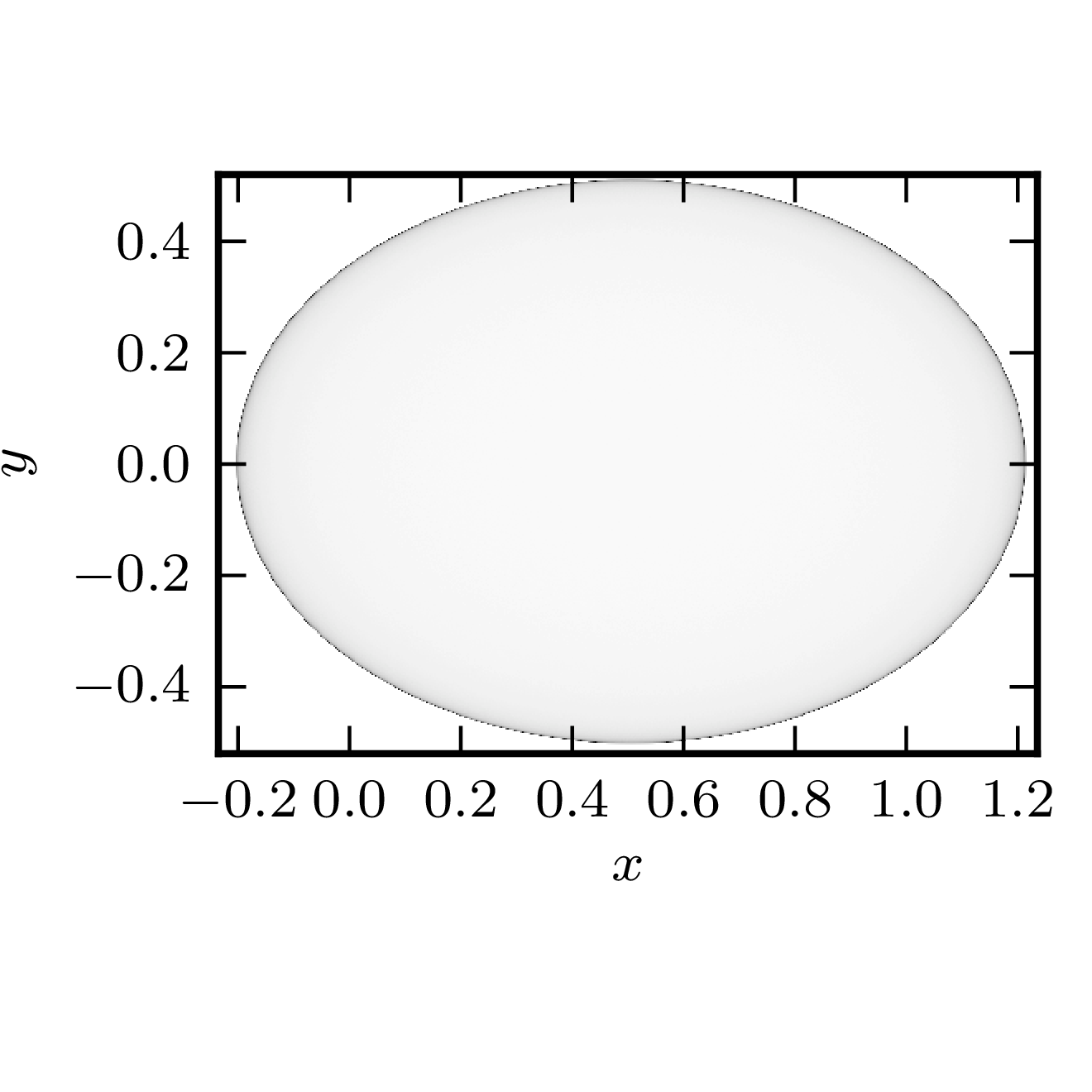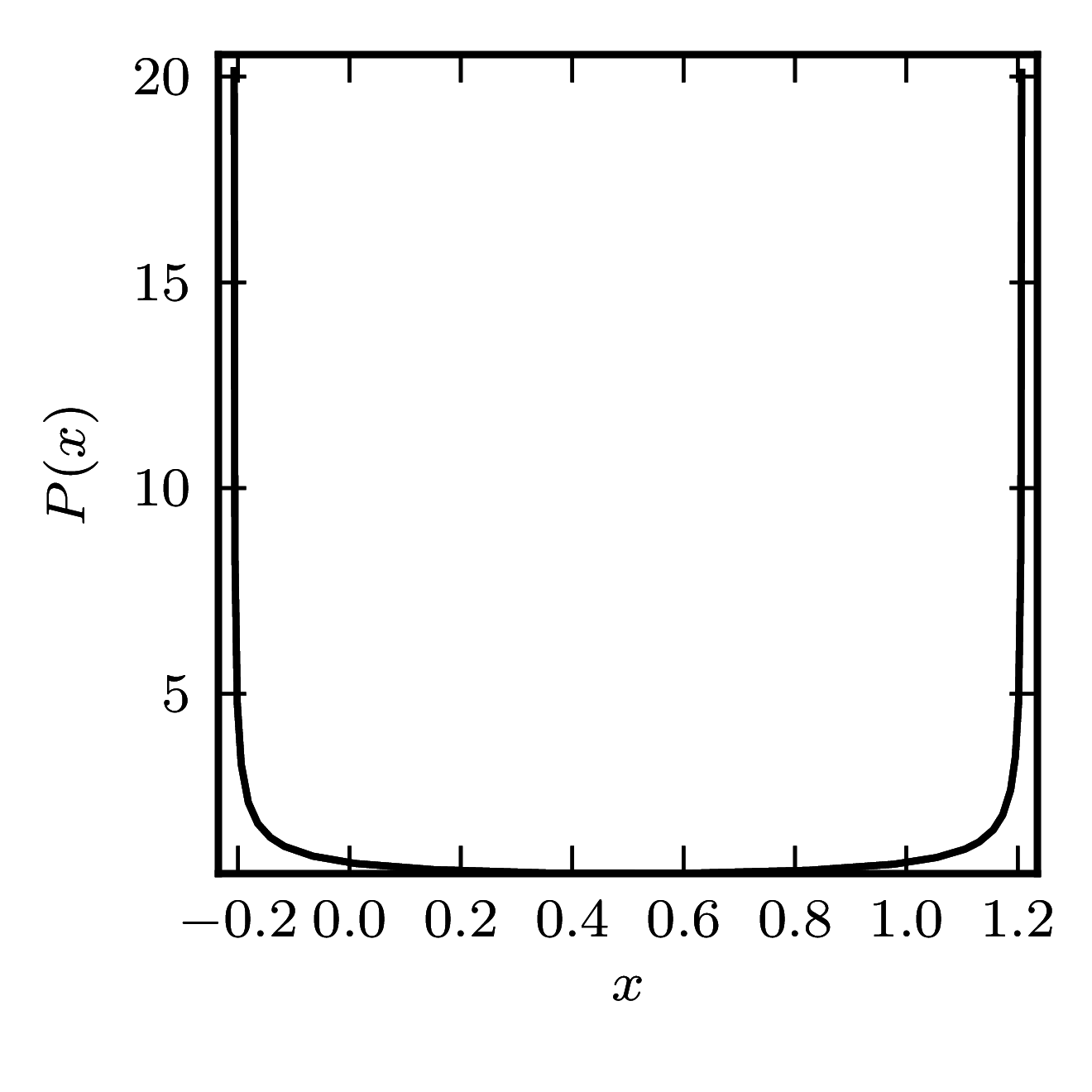# Hermitian matrices

In the case of Hermitian matrices the numerical shadow is a one dimensional distribution.

## Diagonal matrices

### Example 1

The matrix is $\mathrm{diag}(0,6)$

Standard numerical shadow with respect to complex states Numerical shadow with respect to real states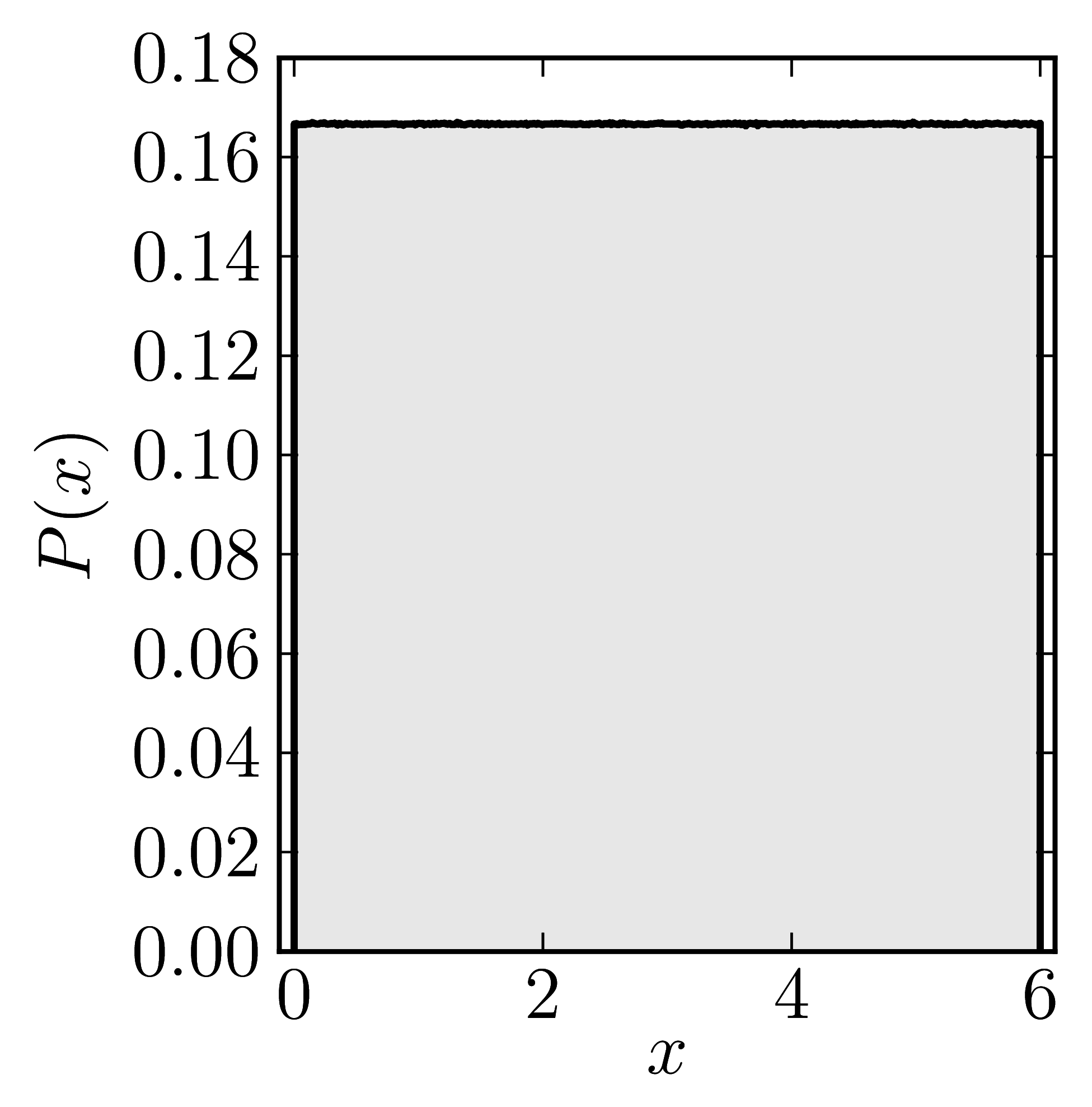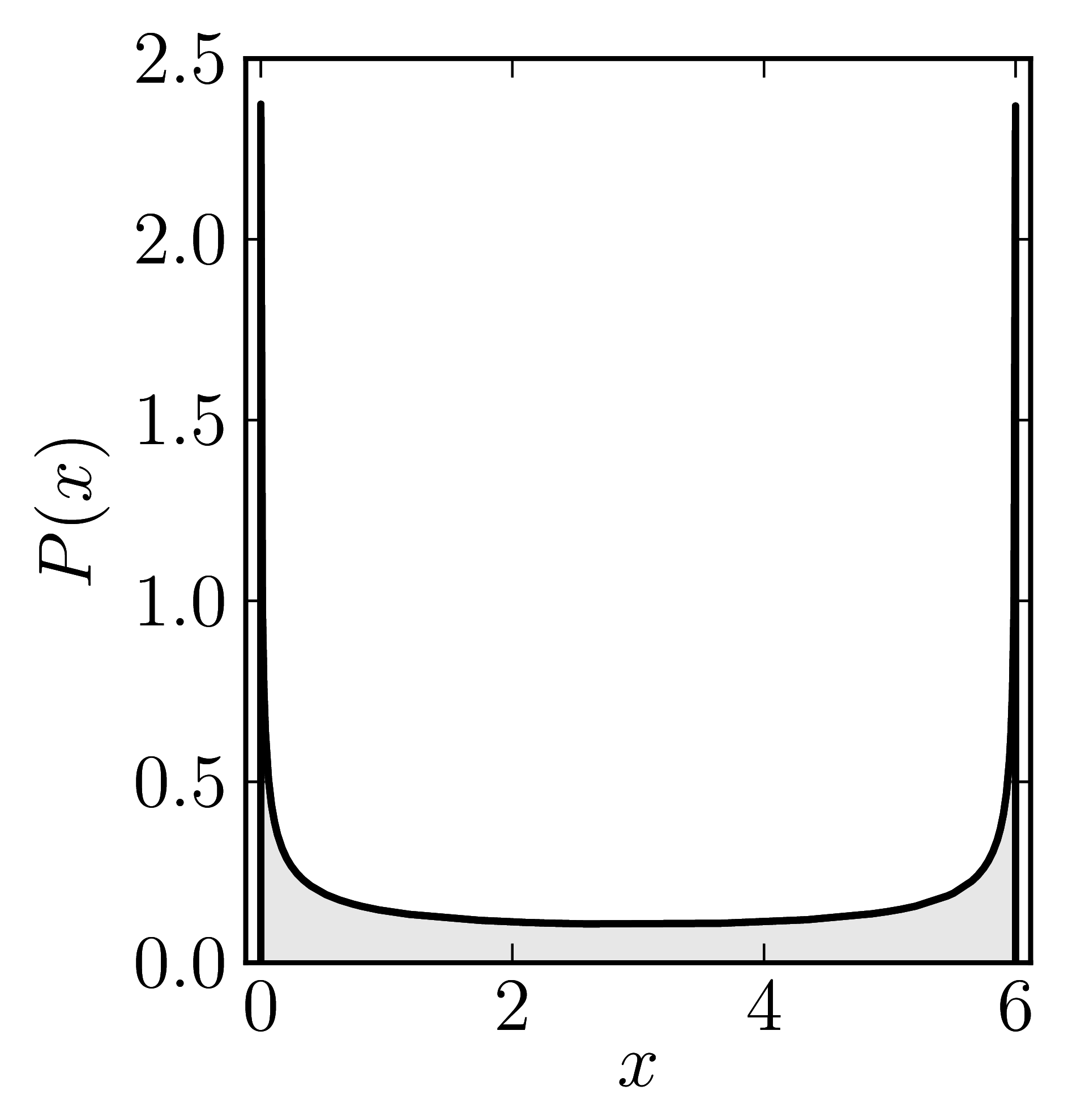# Non-normal matrices

### Example 1

The matrix is $\begin{pmatrix} 0&1\ 0&0 \end{pmatrix}.$ The shadow resembles a physical shadow cast by the hollow sphere made of transparent material when illuminated by a light source at infinity.

Standard numerical shadow with respect to complex states Cross-section of the shadow along the real axis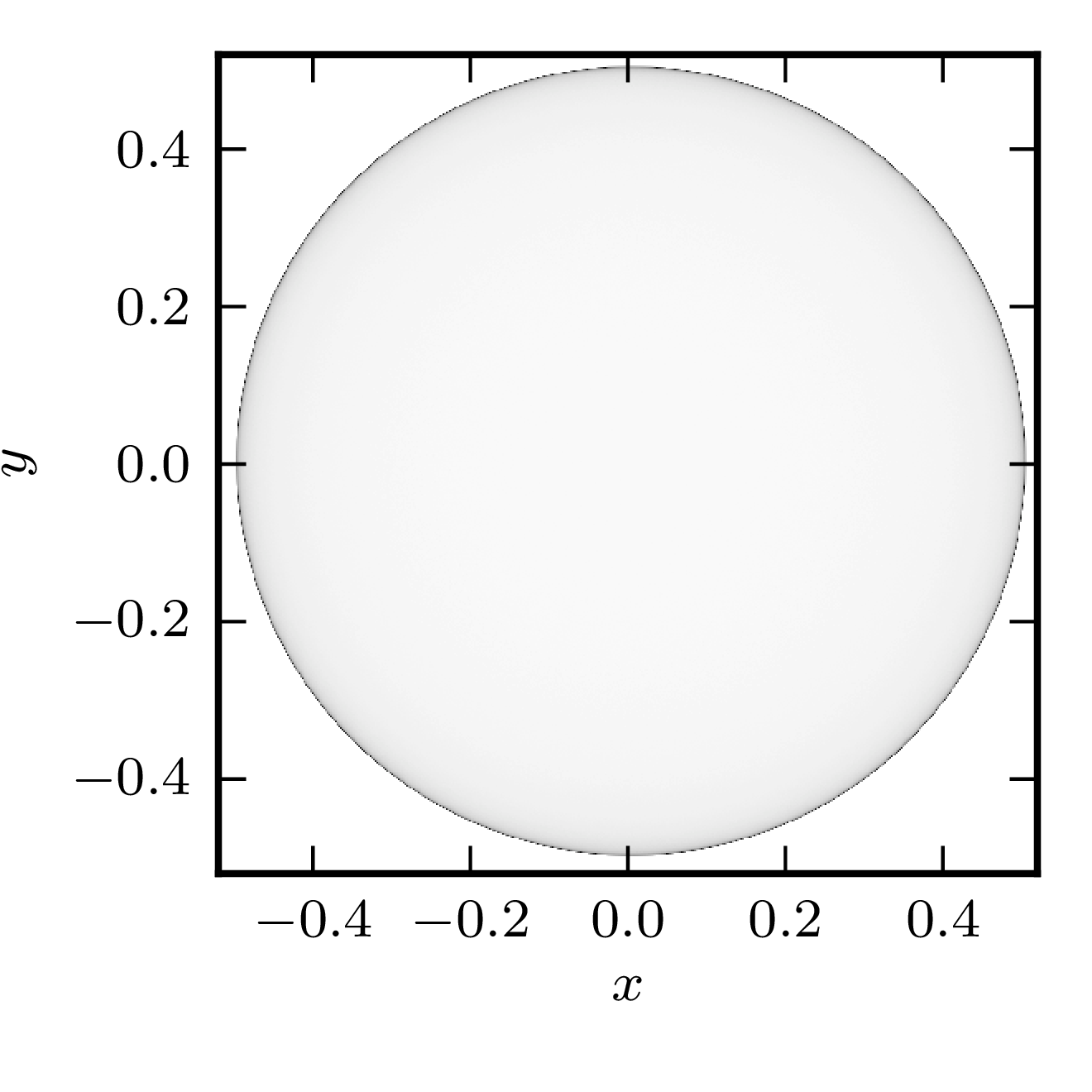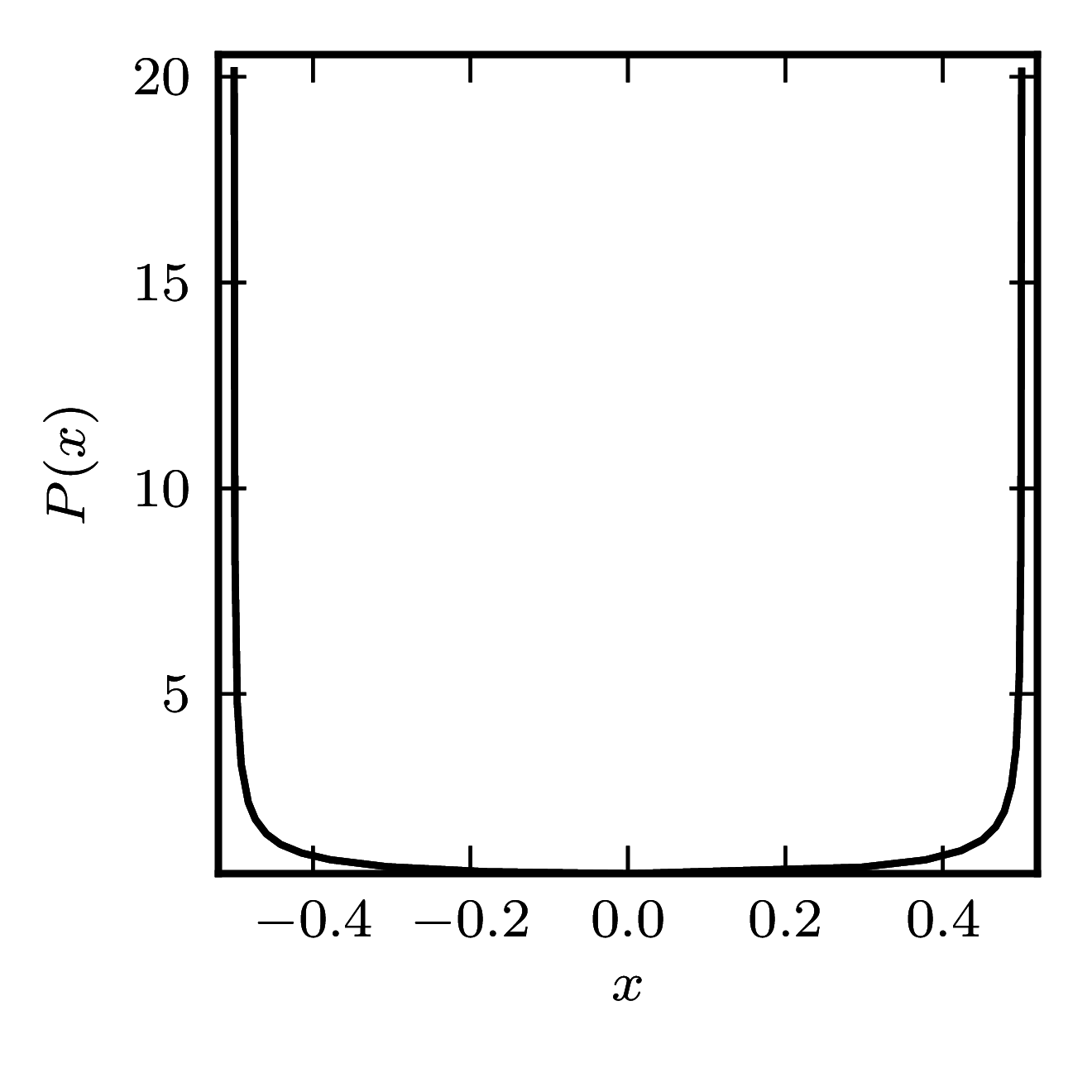### Example 2

The matrix is $\begin{pmatrix} 0&1\ 0&1 \end{pmatrix}.$ The shadow resembles physical shadow cast by the hollow sphere made of transparent material when illuminated by a light source at infinity, but with screen not perpendicular to the light rays.

Standard numerical shadow with respect to complex states Cross-section of the shadow along the real axis